## Introduction

This material is made for the Machine Learning workshop at University of Luxemburg.

Goal?
Through this workshop, we will be able to learn a practical overview of ML modeling workflow and some of the basic skills to get started.

Main package?
We use mlr package. mlr is a wrapper of various available ML algorithms in R (about 150 algorithms); in other words, it is an integrative ML platform. This package solves the issue that each ML package has a slightly different grammar from each other, and it allows users to use a single, simple, & unified way of writing. The tutorial of mlr is also greatly well written and comprehensive from basics to advanced.

Is it enough to know only mlr for studies?
I would say “NO”. mlr is practically useful to know what can be done with ML algorithms, to compare several algorithms, to make a prediction from a trained model. Yet, there are so many attractive functions which are not covered by mlr (esp. for visualization or interpretation).
Nevertheless, when we go deeper, mlr will not satisfy you. So, we need to use some particular packages such as party, which is one of my favorite ML packages (and is run by one of the most active group improving random forest algorithms).

mlr vs caret?
You may know another similar wrapper package: caret. How do they differ? Practically, they differ a bit, but conceptually and practically they are really good alternatives. caret appeared several years earlier, so it has gained far more popularity. There is a super nice summary of the comparison, see this blog by Phillip Probst.

## 1. Settings

##### 1.1. Set the working directory appropriately.
setwd("../R")

##### 1.2. We use a set of R packages.

The packages are automatically installed if needed. Confirm all listed values are TRUE (not FALSE).

package.list = c("party", "mlr", "rpart", "rpart.plot")
tmp.install = which(lapply(package.list, require, character.only = TRUE)==FALSE)
if(length(tmp.install)>0) install.packages(package.list[tmp.install], repos = "http://cran.us.r-project.org")
lapply(package.list, require, character.only = TRUE)

##### 1.3. Let’s read the example dataset.

It contains a response variable (y1) and 16 explanatory variables (x1-x16).

df = data.frame(read.csv("data_example.csv"))
head(df, 5)
##         y1 x1 x2 x3       x4   x5 x6       x7        x8        x9 x10
## 1 2.795473  0  0  0      low    A  B 2.059746  6.774423 10.168996   1
## 2 6.366427  1  1  1     high <NA>  C 1.765568 13.000086 11.528108  NA
## 3 2.452364  0  1  1 moderate    B  A 6.870228  8.136200  3.706351  10
## 4 8.436862  0  0  1 moderate    B  D 3.841037  5.846719  6.986914  NA
## 5 6.987436  1  0  1 moderate    A  C 7.698414        NA 11.607315   7
##         x11 x12 x13       x14       x15      x16
## 1 1.5082529   4   7  8.978635        NA 4.938302
## 2 2.2693967   4   7  6.486254  8.459923 2.918157
## 3 7.4511681   8   4  7.511756  7.562344 9.600311
## 4 0.5059238   7   6 10.687636  5.112040 6.294878
## 5 5.9399330   4   4  7.555021 11.706717 7.593479

## 2. Overview of ML workflow

#### In this seciton, we learn what can be typically done using a ML.

• Defining a task, selecting an algorithm, and training it to build a model (regression)
• Assessing the built model performance
• Fine-tuning the model
• Interpreting the results (variable importance and partial dependence plots)

#### 2.1. Defining a task, selecting an algorithm, and training it to build a model (case study: regression)

##### 2.1.1. Dataset: training and testing

First of all, let’s split the dataset into two subsets.
One portion is used for traning an algorithm, and the other is used for evaluating the built model performance. Note that there are several ways to conduct performance assessment (e.g. k-fold cross validation, out-of-bag). There are some preset functions in mlr. We use 90% of the samples for training, and the rest is used for performance assessment.

train.set = sample(1:nrow(df), size = nrow(df)*0.9, replace = F)
test.set  = setdiff(1:nrow(df),train.set)

##### 2.1.2. Task, Learner, Train & Predict

As a typical workflow, we do the followings:

1. Setting up a task (i.e. what to do?)
2. Specifying an algorithm (i.e. which tool to use?)
3. Traning the algorithm with the task (i.e. model building)
4. Assessing model performance (i.e. how well the algorithm learned from the data?)
regr.task = makeRegrTask(data = df, target = "y1")
regr.lrn = makeLearner("regr.cforest")
regr.mod = train(regr.lrn, regr.task, subset = train.set)
regr.mod
## Model for learner.id=regr.cforest; learner.class=regr.cforest
## Trained on: task.id = df; obs = 270; features = 16
## Hyperparameters:
task.pred = predict(regr.mod, task = regr.task, subset = test.set)
performance(task.pred, measures=list(mse, rsq))
##       mse       rsq
## 14.363890  0.324622
plot(as.data.frame(task.pred)[,c("truth","response")])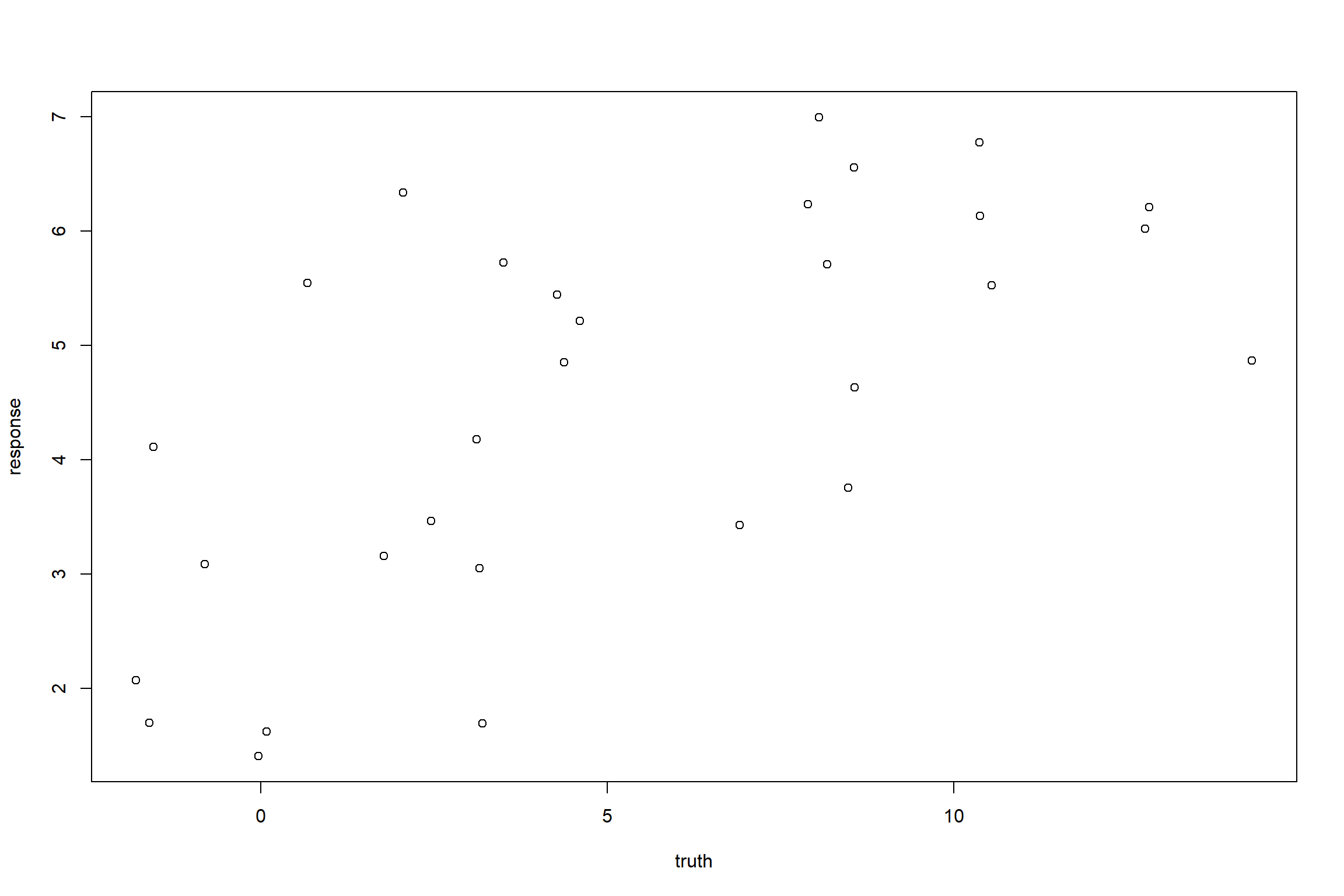Note that we use the conditional infrerence forest (cforest) algorithm here, but anything else can be used. There are several measures to evaluate model performance. Here, we checked Mean Squared Error (mse) and R-squared (rsq), but what to report depends on discpiline. See:

listMeasures(regr.task)
##   "featperc"    "mae"         "mape"        "medse"       "msle"
##   "rae"         "spearmanrho" "timeboth"    "timetrain"   "rmsle"
##  "timepredict" "medae"       "sse"         "expvar"      "kendalltau"
##  "rmse"        "mse"         "rrse"        "sae"         "rsq"
##  "arsq"

#### 2.2. Fine-tuning the model

Many algorithms have so-called “hyperparameters”, which are NOT estimated from the data (unlike the parameters of a statistical model). A user needs to pre-set these values, and they influence on model performance.

Here, we see the list of hyperparameters of cforest algorithm that can be manually changed:

getParamSet(regr.lrn)
##                    Type len        Def
## ntree           integer   -        500
## mtry            integer   -          5
## replace         logical   -      FALSE
## trace           logical   -      FALSE
## fraction        numeric   -      0.632
## testtype       discrete   - Univariate
## mincriterion    numeric   -          0
## minsplit        integer   -         20
## minbucket       integer   -          7
## stump           logical   -      FALSE
## nresample       integer   -       9999
## maxsurrogate    integer   -          0
## maxdepth        integer   -          0
## savesplitstats  logical   -      FALSE
##                                                  Constr Req Tunable Trafo
## ntree                                          1 to Inf   -    TRUE     -
## mtry                                           1 to Inf   -    TRUE     -
## replace                                               -   -    TRUE     -
## trace                                                 -   -   FALSE     -
## fraction                                         0 to 1   Y    TRUE     -
## teststat                                       quad,max   -    TRUE     -
## testtype       Bonferroni,MonteCarlo,Univariate,Test...   -    TRUE     -
## mincriterion                                   0 to Inf   -    TRUE     -
## minsplit                                       1 to Inf   -    TRUE     -
## minbucket                                      1 to Inf   -    TRUE     -
## stump                                                 -   -    TRUE     -
## nresample                                      1 to Inf   -    TRUE     -
## maxsurrogate                                   0 to Inf   -    TRUE     -
## maxdepth                                       0 to Inf   -    TRUE     -
## savesplitstats                                        -   -   FALSE     -

That’s a lot!! Yet, in the case of random forest algorithms, typically, the most influential hyperparameters are the number of tree models (ntree) and the number of random sampling from the set of the predictors (mtry).
For regression, mtry is often set to the square root of the number of predictors for empirical reasons.

We attempt to find a combination of these hyperparameters which produce the best performance.
In the following, the function makeParamSet prepares several different parameter combinations of ntree and mtry. As they are both integer, we use makeIntegerParam. Both upper and lower boundaries are set.

ps = makeParamSet(
makeIntegerParam("ntree", lower = 1, upper = 100),
makeIntegerParam("mtry", lower =  1, upper = 10)
)

Then, we set the resolution of the search (interval of parameter value, how many times cross validation is conducted). Resolution and iterations should be ideally large enough, but it they are too large, it takes too much time.

ctrl = makeTuneControlGrid(resolution = 10)
rdesc = makeResampleDesc("CV", iters = 5)
tune.cforest = tuneParams(regr.lrn, task = regr.task, resampling = rdesc, par.set = ps, control = ctrl)
plotHyperParsEffect(generateHyperParsEffectData(tune.cforest), x = "ntree", y = "mtry", z = "mse.test.mean",
plot.type = "heatmap")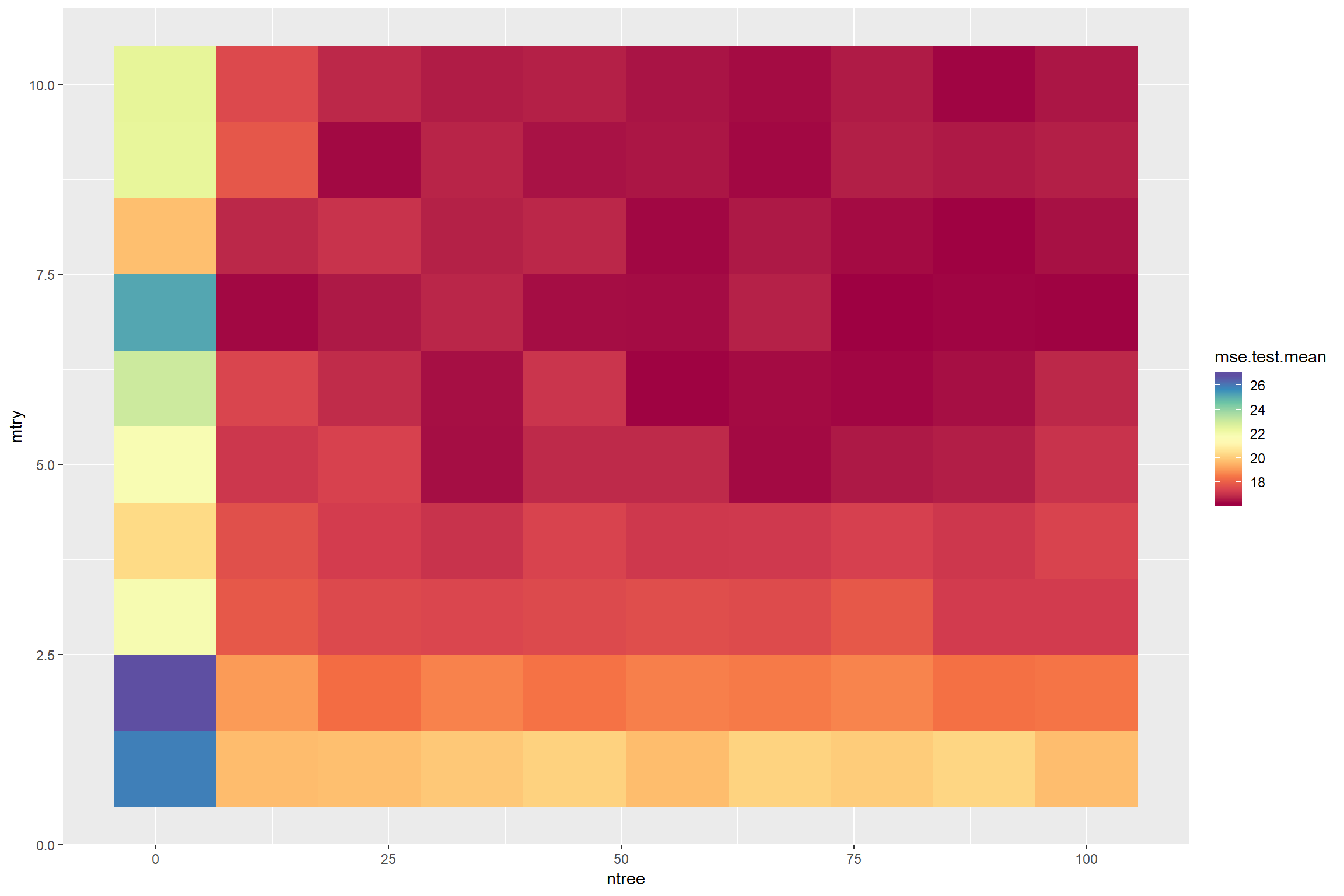tune.cforest$x ##$ntree
##  78
##
## $mtry ##  7 We find (a kind of) the best performing hyperparameters. Yet, as we see, not always we find the single best combination, and testing too large parameter combinations take time really much. Our dataset is very small, so this can be readily done, but sometimes it is really hard to tune an algorithm. An important thing is to find a minimally satisfactory performance set. In case of random forest, there are many packages having function of random forest, but the package “ranger” is the fastest one in R. FYI. regr.lrn.best = setHyperPars(makeLearner("regr.cforest"), ntree = tune.cforest$x$ntree, mtry = tune.cforest$x$mtry) regr.mod.best = train(regr.lrn.best, regr.task, subset = train.set) #### 2.3. Interpreting the results (variable importance and partial dependence plots) OK, (let’s say) we tuned the model(or, we confirmed the performance seems good enough with the given parameter set). Variable importance What we do next is to interpret the model. Opening the black-box. Firstly, we evaluate which predictors are the important ones for predicting the response variable. Variable importance measure (VIMP) is estimated for each predictor. Note that there are several algorithms proposed to estimate VIMP. Sometimes, selecting an appropriate one is required. vimp = unlist(getFeatureImportance(regr.mod.best)$res)
barplot(vimp)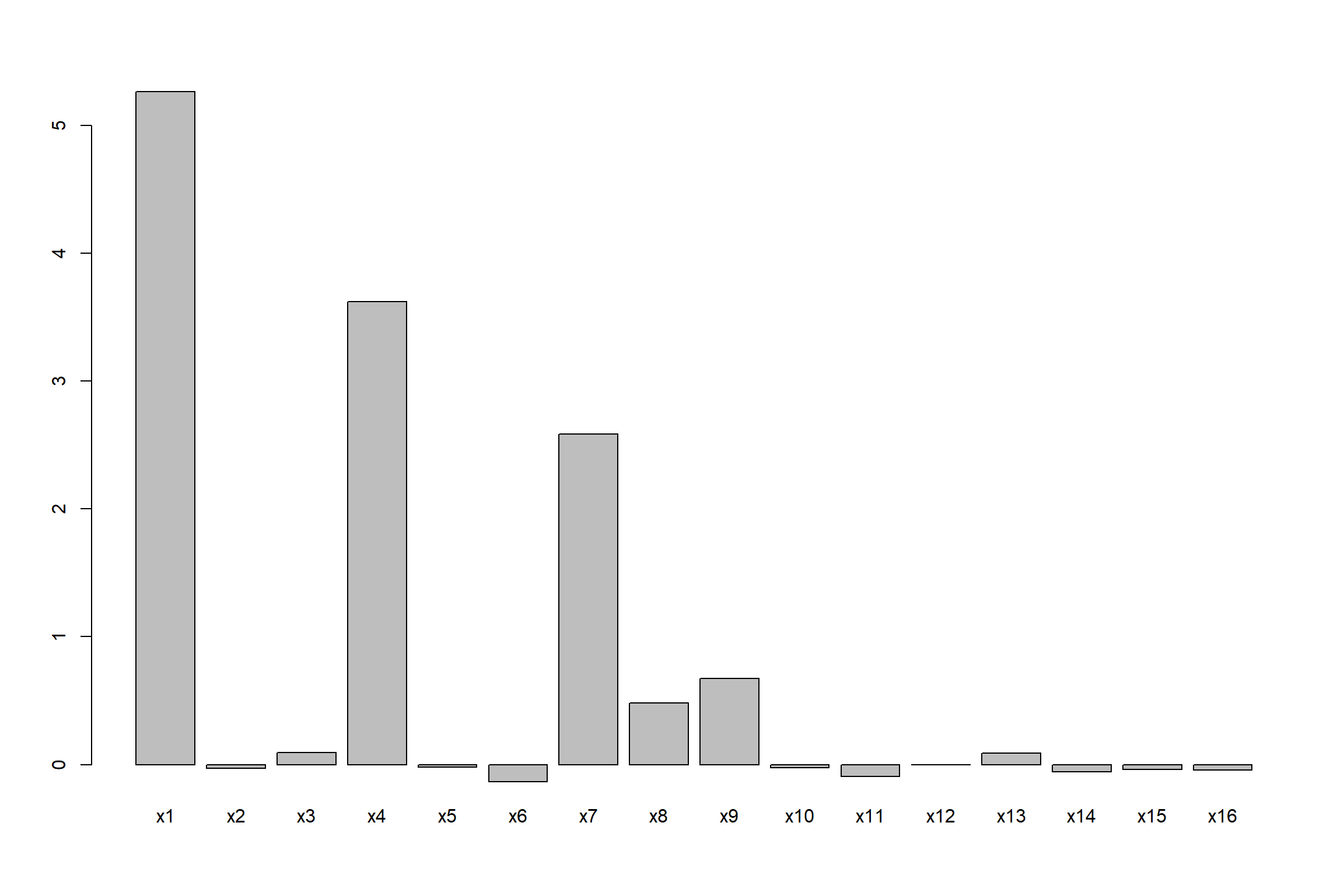Now we see that only a few variables strongly contributes to the model. x1, x4, x7, x8, x9. It is a sort of effect size in statistics. It typically expresses how much each predictor contributes to model performance.
Why do some have a negative important score? It means that these predictors are just noisy variables and if further fine-tuning is preferred, they should be omitted. This is related to feature selection, but this is not covered in this workshop.

Partial dependence plots Then, we want to see HOW each predictor is associated with the response variable: positively or negatively. In addition, we may want to see if there is a nonlinear pattern and threshold value. We use a popular one, partial dependence plots.

pdp.all = generatePartialDependenceData(regr.mod.best, regr.task, individual = F)
## Loading required package: mmpf
plotPartialDependence(pdp.all)
## Warning in melt.data.table(data.table(obj$data), id.vars = colnames(obj ##$data)[!colnames(obj$data) %in% : 'measure.vars' [x1, x2, x3, x4, ...] ## are not all of the same type. By order of hierarchy, the molten data ## value column will be of type 'double'. All measure variables not of type ## 'double' will be coerced too. Check DETAILS in ?melt.data.table for more on ## coercion.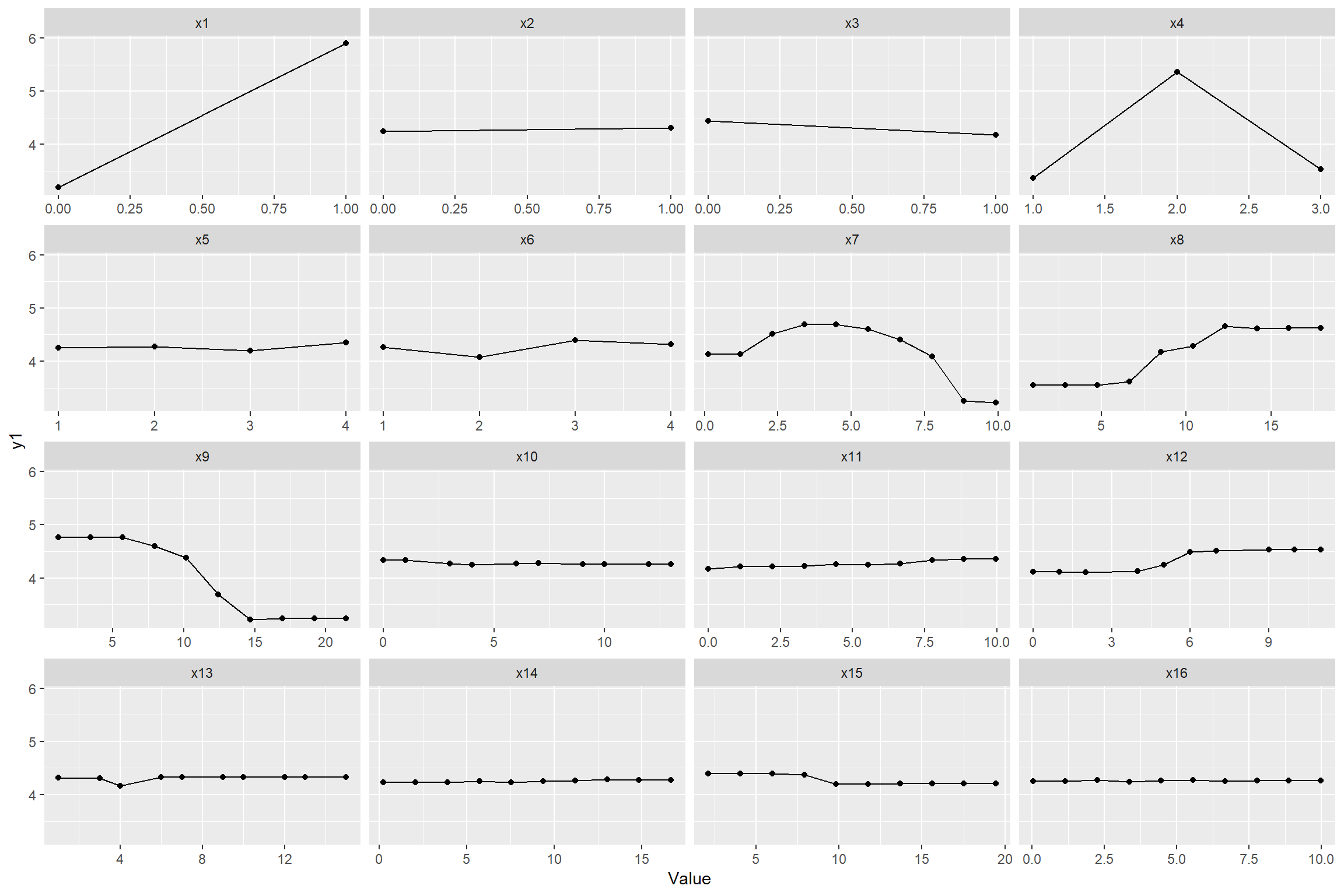We can observe that x7 has a unimodal pattern, x8 & x9 show a threshold nonlinear pattern. Note that categorial variables (e.g. x1-x5) are plotted with points connected with a line, but ideally, they are better expressed as barplots (no function embedded to mlr). … If we want to check more (e.g. variable interaction), it is really worth reading the book, interpretable Machine Learning ## 3. Introduction to decision tree algorithms #### In this seciton, we learn some decision tree algorithms. • CART (Breiman’s decision tree) • conditional inference tree (Hothorn’s decision tree) We do not use mlr hereafter. Even though mlr is useful generalization, many packages are well specialized for some particular algorithms and they do have far more enhanced functions. ### 3.1. CART ##### CART (classification and regression tree) CART was proposed by Breiman et al. (1984). Although some decision tree algorithms had already been proposed earlier than their work, their work made tree algorithms useful. The key finding was so-called “pruning” method, which takes the balance between complexity and accuracy. Formula Here make a regression formula, y1 is regressed by the set of predictors x1-x16. for the formula it uses “+” among predictors but it does not mean they are additive. # creating formula formula.1 = as.formula(paste("y1", paste(colnames(df)[2:length(colnames(df))], collapse=" + "), sep=" ~ ")) print(formula.1) ## y1 ~ x1 + x2 + x3 + x4 + x5 + x6 + x7 + x8 + x9 + x10 + x11 + ## x12 + x13 + x14 + x15 + x16 Modeling For regression use method=“anova”; for classification, use method=“class” # regression cart.1 = rpart(formula.1, data=df, method="anova",control=rpart.control(minsplit=10, cp=0.001))  Option Description method class method for a classification tree anova method for a regression tree control minsplit minimum number of observations in a node before attempting a split cp cost complexity factor. a split must decrease the overall lack of fit by a factor of cp before being attempted. Hyperparameter Remember that you can change hyperparameters! Let’s check how cp affects model performance. Firstly, CART algorithm splits data as much as possible, unless minsplit is met. At the top, x7 at the value of 8.2 was selected to split the dataset into two. Each of the circles (terminal node) represents an expected value of the response variable, conditioned by a set of predictors and their threshold values. prp(cart.1) # plot with rpart.plot package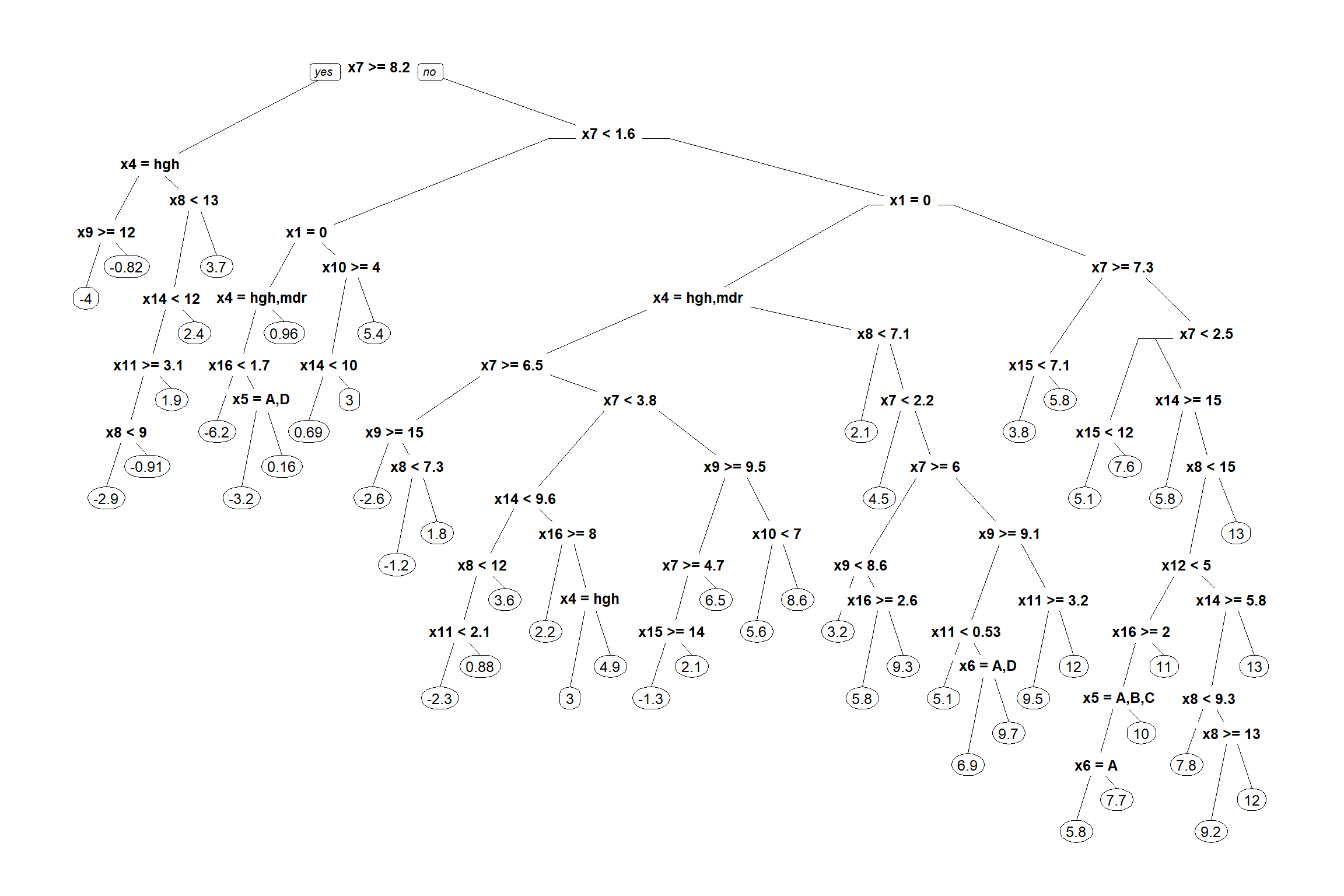Overfitting Yet, the tree is unnecessary complex and overfits to the data. So, we want to simplify the tree structure by pruning (cutting) some (too detailed) brunches, while keeping model performance. Cross-validation is the key to find how much we can prune the tree.  rsq.rpart(cart.1) #plot approximate R-squared and relative error for different splits (2 plots). labels are only appropriate for the "anova" method. ## ## Regression tree: ## rpart(formula = formula.1, data = df, method = "anova", control = rpart.control(minsplit = 10, ## cp = 0.001)) ## ## Variables actually used in tree construction: ##  x1 x10 x11 x12 x14 x15 x16 x4 x5 x6 x7 x8 x9 ## ## Root node error: 6876/300 = 22.92 ## ## n= 300 ## ## CP nsplit rel error xerror xstd ## 1 0.1478943 0 1.00000 1.00694 0.078257 ## 2 0.0897085 2 0.70421 0.78959 0.062994 ## 3 0.0749399 3 0.61450 0.73614 0.059929 ## 4 0.0341506 4 0.53956 0.60281 0.049835 ## 5 0.0312389 5 0.50541 0.59951 0.049501 ## 6 0.0229716 6 0.47417 0.59038 0.047721 ## 7 0.0183607 7 0.45120 0.60974 0.050744 ## 8 0.0169285 9 0.41448 0.60015 0.048792 ## 9 0.0165060 11 0.38062 0.59527 0.048570 ## 10 0.0120166 14 0.33111 0.58872 0.048776 ## 11 0.0098657 15 0.31909 0.59482 0.048535 ## 12 0.0084060 16 0.30922 0.62882 0.053774 ## 13 0.0080542 17 0.30082 0.65611 0.055260 ## 14 0.0075239 18 0.29276 0.67160 0.055586 ## 15 0.0072233 19 0.28524 0.69647 0.056368 ## 16 0.0066753 20 0.27802 0.70776 0.057399 ## 17 0.0065915 21 0.27134 0.70941 0.057885 ## 18 0.0064820 23 0.25816 0.71025 0.057870 ## 19 0.0056757 24 0.25168 0.70870 0.058253 ## 20 0.0052401 25 0.24600 0.70887 0.058055 ## 21 0.0052287 26 0.24076 0.70812 0.058406 ## 22 0.0047481 31 0.21462 0.69983 0.058573 ## 23 0.0045622 32 0.20987 0.70137 0.058595 ## 24 0.0043403 33 0.20531 0.71030 0.059665 ## 25 0.0041641 34 0.20097 0.71258 0.059716 ## 26 0.0040764 35 0.19680 0.70921 0.059540 ## 27 0.0037831 36 0.19273 0.71342 0.059628 ## 28 0.0035694 37 0.18894 0.70696 0.059367 ## 29 0.0035456 38 0.18537 0.70634 0.058972 ## 30 0.0032837 39 0.18183 0.70276 0.058853 ## 31 0.0032120 40 0.17854 0.70091 0.058173 ## 32 0.0032033 41 0.17533 0.70091 0.058173 ## 33 0.0030934 42 0.17213 0.70126 0.058211 ## 34 0.0030602 43 0.16903 0.70157 0.058199 ## 35 0.0025556 44 0.16597 0.70198 0.059045 ## 36 0.0018902 45 0.16342 0.69429 0.058829 ## 37 0.0016425 46 0.16153 0.69260 0.060802 ## 38 0.0013018 47 0.15989 0.69640 0.061539 ## 39 0.0012938 48 0.15858 0.69700 0.061536 ## 40 0.0012298 50 0.15600 0.69935 0.061919 ## 41 0.0010000 51 0.15477 0.69935 0.061919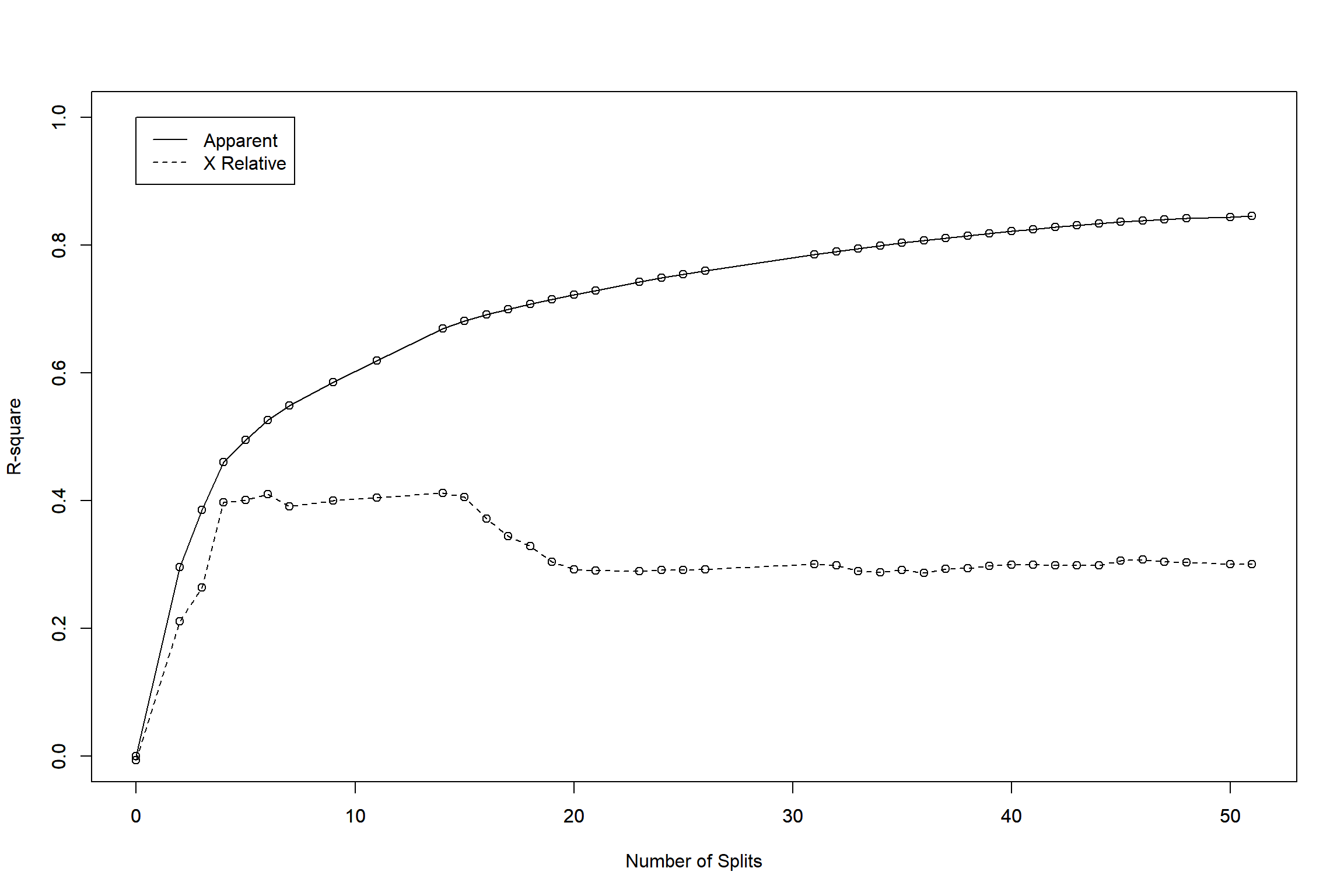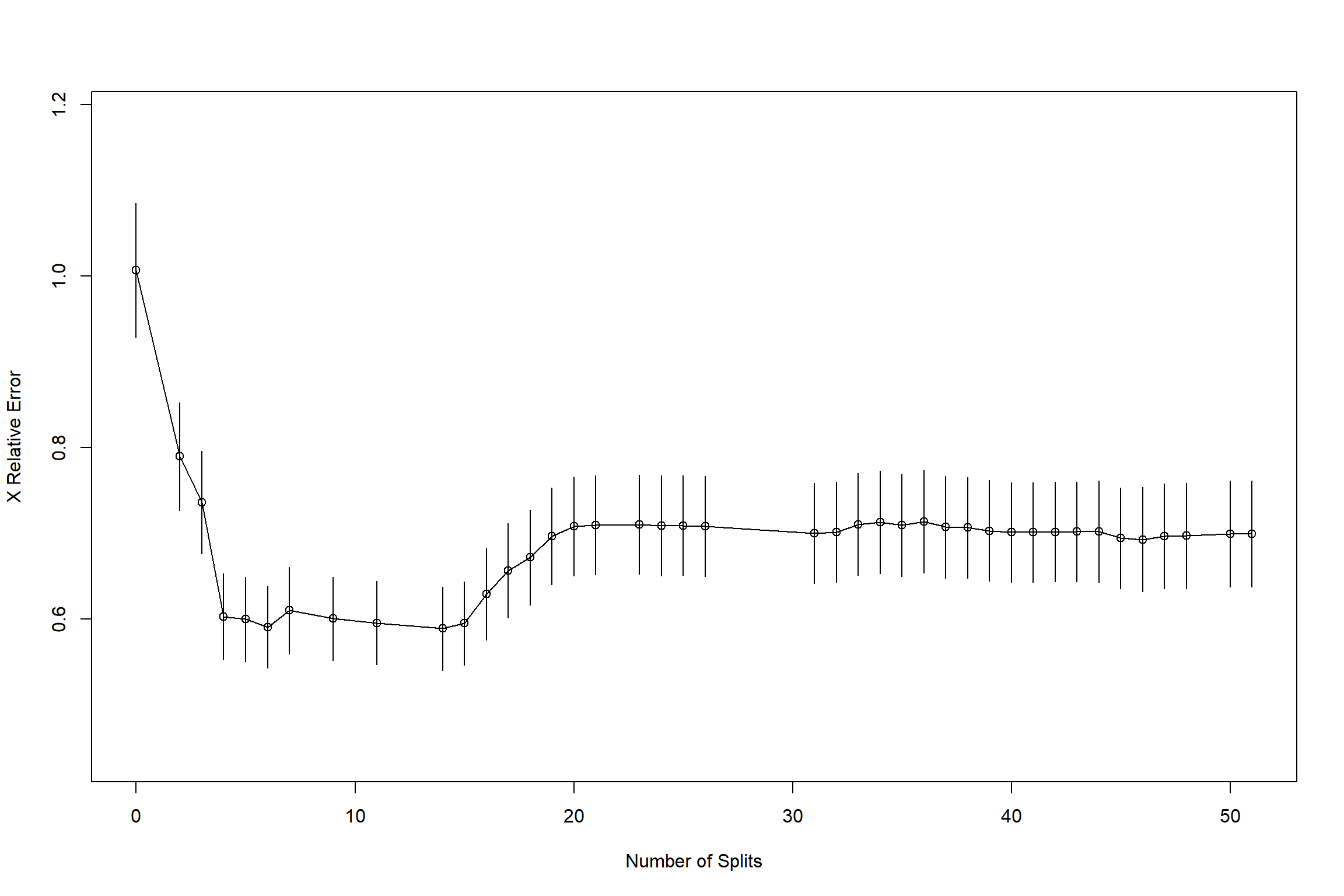cp.best = cart.1$cptable[which(cart.1$cptable[,"xerror"]==min(cart.1$cptable[,"xerror"])),"CP"]

Now, we found cp=0.0120166 the best for balancing complexity and accuracy (note that the performance even improved by reducing overfitting!). Let’s compare the best and original tree structures.

# regression (y1) and classification (y2)
cart.1.best = rpart(formula.1, data=df, method="anova",control=rpart.control(minsplit=10, cp=cp.best))

par(mfrow=c(1,2))
prp(cart.1.best)
prp(cart.1)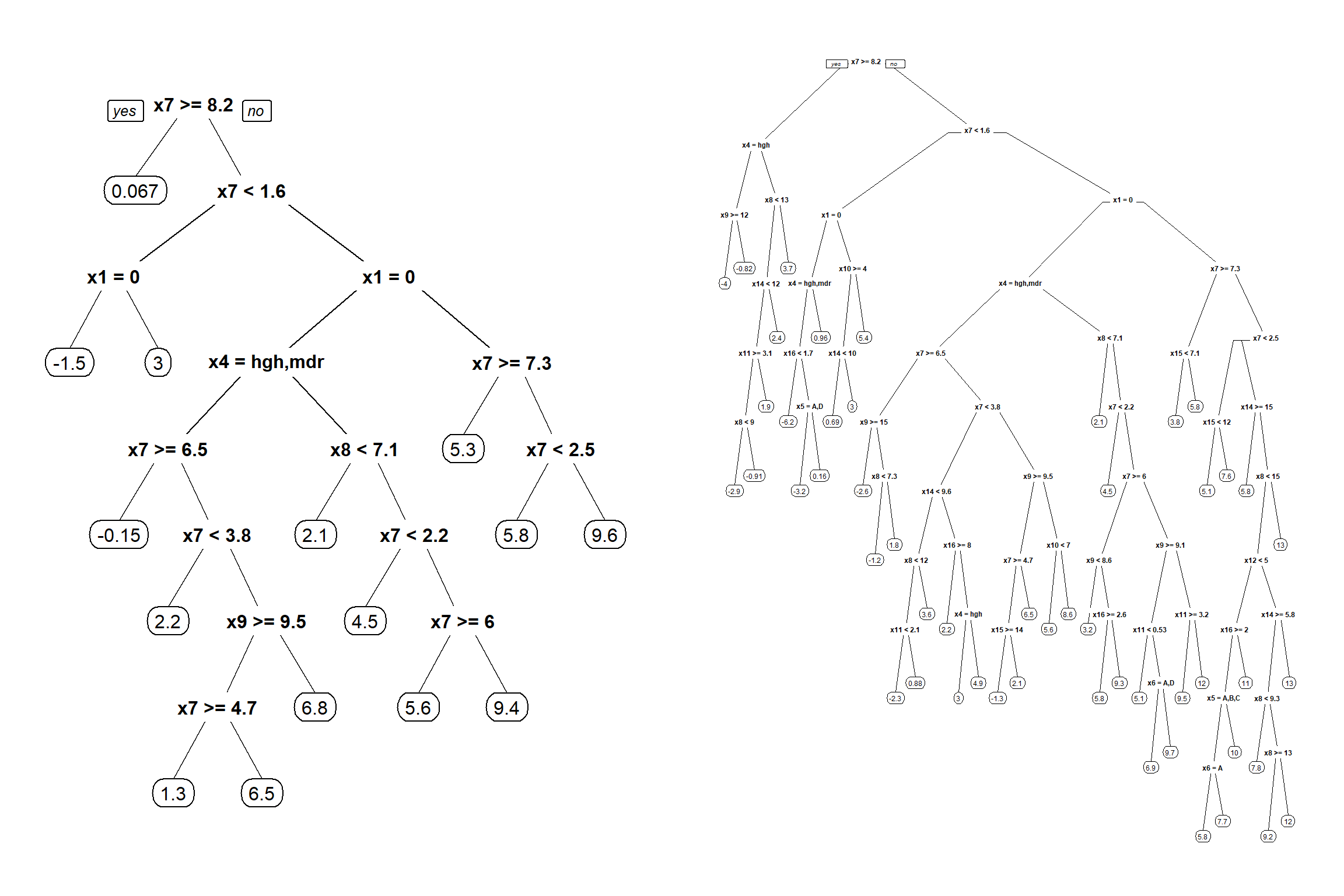You can also play with changing minsplit. This is another hyperparameter.

### 3.2. Conditional inference tree

Conditional inference tree is another tree-based algorithm developed relatively recently by Hothorn et al. (2006). They found that, if the data must be split more or not can be determined based on statistical significance, and by doing so the structure of a tree can be much simplified while the performance does not significantly reduce from the model selected based on CART CV-technique.
We use party package:

ctree.1 = ctree(formula.1, df, control = ctree_control(testtype = "Bonferroni", mincriterion = 0.95, minsplit = 10, minbucket = 7))
plot(ctree.1)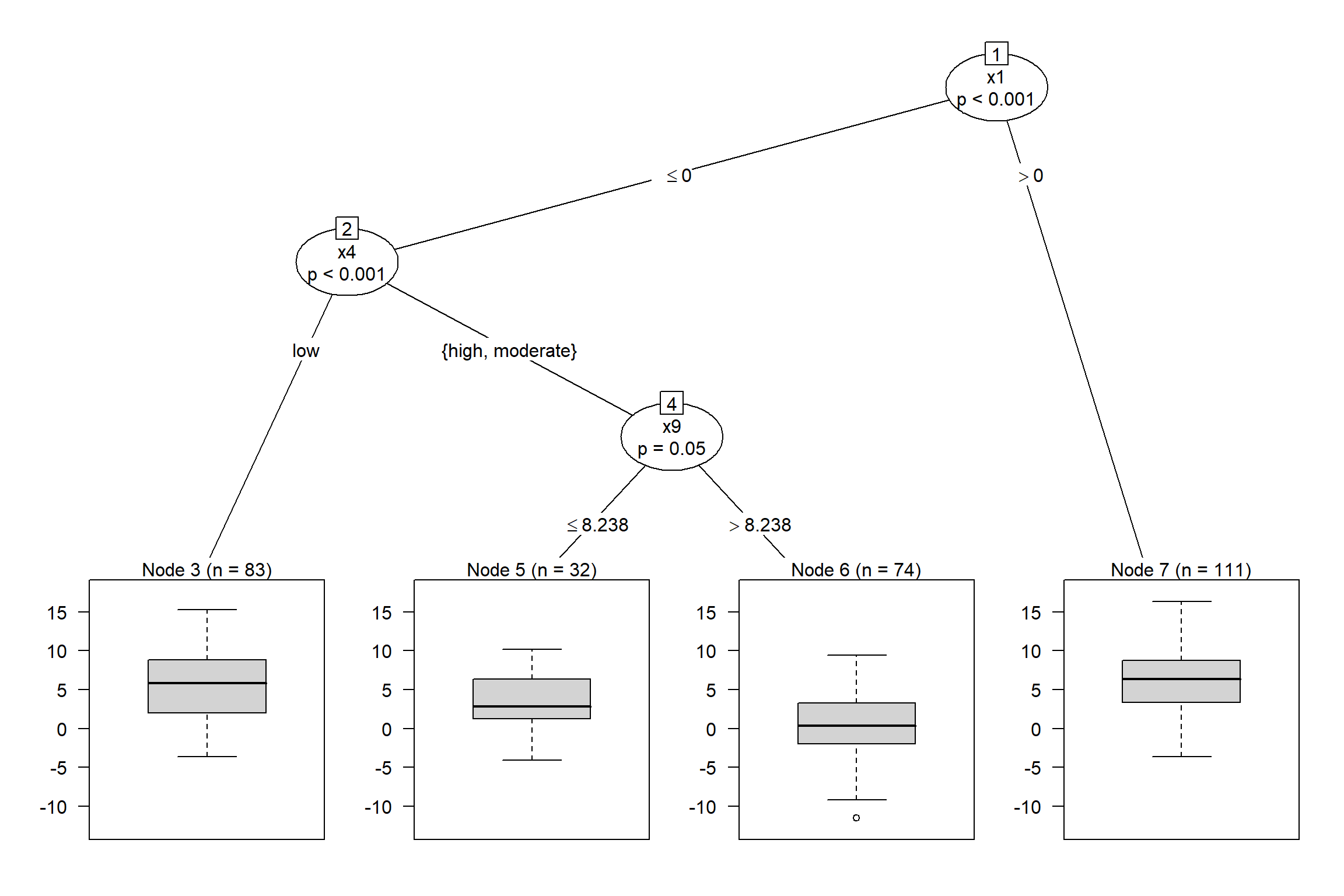If testtype is “Univariate”, p-value correction is not peformed, so more predictors can appear. If implementing correction or not is always difficult, as it is a trade-off of Type 1 & 2 error.

ctree.2 = ctree(formula.1, df, control = ctree_control(testtype = "Univariate", mincriterion = 0.95, minsplit = 10, minbucket = 7))
plot(ctree.2)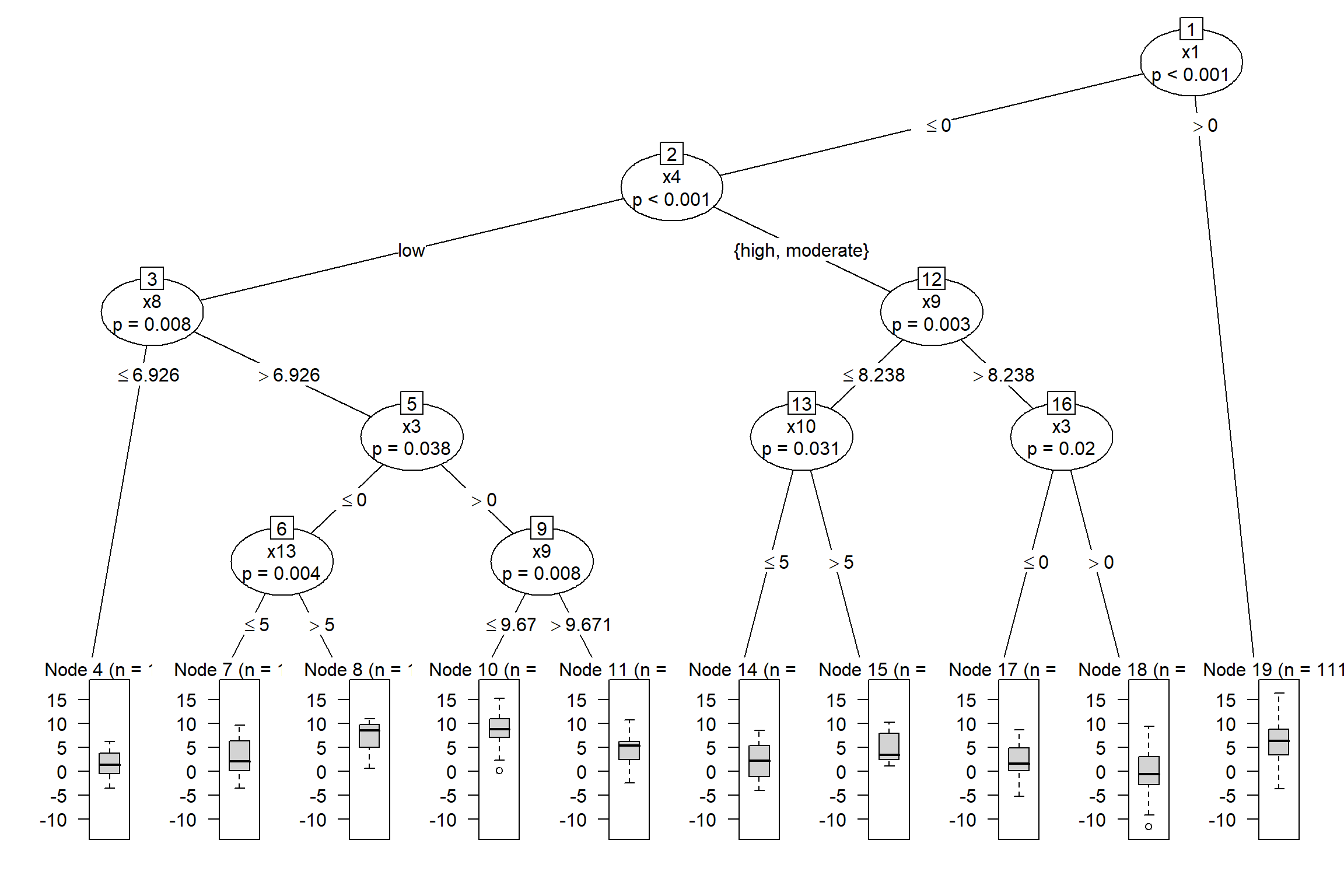## 4. Concluding Remarks

Well done, that’s it!! Through this workshop, we have learned the overview of ML workflow as well as some specific (my favorite) decision tree algorithms. I have to admit that this course did not cover many other important algorithms such as k-NN, artificial neural net, support vector machine, deep learning…
Nevertheless, I believe that we now have a good intuition what ML is.
Hope many of you will continue learning ML. There are plenty of useful Youtube videos available, as well as free e-books, so you should be able to deepen your knowledge further!!

by Masahiro Ryo